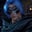Related Tags

node
communitycreator

# What is a balanced binary tree?Gutha Vamsi Krishna

A height-balanced binary tree is a binary tree in which the left and right subtrees of every node differ in height by no more than 1.

This means that if even one node has a height difference of more than 1 for the left and right subtrees, then it is not a balanced binary tree.

### Balanced binary tree

To check whether or not the tree above is balanced, we need to check if every node is balanced.

#### Node 3

The height of the left subtree for node 3 is 1 since it only has one left child,9, and the height of the right subtree for node 3 is 2.

We can calculate the height for the right subtree as $20 --- 15$ or $20 --- 7$, as it is the same.

The difference between the left and right subtrees for node 3 is 1, so this node is balanced.

Now, we need to check the remaining nodes.

#### Node 9

There is no left subtree or right subtree for node 9, so the difference between the height of the left and right subtrees for node 9 is 0. Since 0 is less than 1, node 9 is balanced.

#### Node 20

The height of the left subtree is 1 for node 20 since it has only one node, 15. The height of the right subtree for node 20 is 1 since it has one node, 7.

Since the difference between the subtrees is 0, node 20 is balanced.

#### Node 15

There is no left subtree or right subtree for node 15, so the difference between the height of the left and right subtrees for node 15 is 0. Since 0 is less than 1, node 15 is balanced.

#### Node 7

There is no left or right subtree for node 7, so the difference between the height of the left and right subtrees is 0. Since 0 is less than 1, node 7 is balanced.

### Conclusion

Since every node is balanced in this binary tree, then it is a balanced binary tree.

### Unbalanced binary tree

To check if the binary tree below is balanced or not, we will start with node 1.

• The height of the left subtree for node 1 is 3.

• The height of the right subtree for node 1 is 1.

• Since the absolute difference between the subtrees is 2, node 1 is not balanced.

There is no need to check the remaining nodes.

If even one node in a binary tree is not balanced, then the entire binary tree is not a balanced binary tree.

Therefore, this binary tree is an unbalanced binary tree.

RELATED TAGS

node
communitycreator

CONTRIBUTORGutha Vamsi Krishna
RELATED COURSES

View all Courses

Keep Exploring

Learn in-demand tech skills in half the time